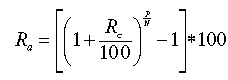# Annualized Rate

Financial analysis Print Email

Annualized rate is a rate of return for a given period that is less than one year, but it is calculated as if the rate were for a full year. It is essentially an estimated annual rate of return that is mathematically extrapolated. The annualized rate is calculated by multiplying the change in the rate of return in one month by 12 (or one quarter by four) to obtain the rate for the year. The annualized return is calculated on a time-weighted basis.

For example, if one month's rate of return is 0.21% and the next month's rate is 0.29%, the change in the rate of return from one month to the next is 0.08% (0.29-0.21). The annualized rate of return is 0.08% x 12 = 0.96%. However, the more accurate way is to calculate the geometric average rate of return.

The annualized (geometric) rate of return is calculated as follows:Where Equals Ra Annualized rate of return Rc Cumulative rate of return P Periodicity (number of time periods in a year: 4 for quarterly data, 12 for monthly data, 365 for days) N Number of time periods in observed diapason

## How to Calculate Annualized Rate of Return

I started the Prudent Portfolio with \$10,000 almost 65 days ago. As of this morning, it had an account balance of \$11,025, with a total gain of \$1,025. If that were the gain for the year, it would be a 10.25% return for the year. But it isn't. It's the return for 65 days. So how do you annualize that number to get a return for a year? That's easy enough. Here's the formula:

((1 + Rate of Return) ^ (365/65)) - 1

365 - days in whole year

The Rate of Return is 10.25% or 0.1025

So, the formula looks like this:

((1 + 0.1025) ^ (365/65) - 1

((1.1025) ^ 5.615385) - 1

0.729705 or 72.97%

You can now see the annualized return on your investment. It may decrease over the course of the year because this high rate cannot necessarily be maintained without very detailed market analysis combined with timely buying and selling of stocks.

## In what fields is the annualized rate applied?

Annualized rate is used in various fields and is a way to express a rate of change, return, or growth on an annual basis. Some common examples of where annualized rate is used include:

Finance: In finance, annualized rate is often used to express the return on an investment over a period of time. For example, the annualized return on a stock or bond over a period of time, such as a year, can be calculated by taking the total return for that period and expressing it as a percentage of the initial investment.

Economics: In economics, annualized rate is used to express the rate of inflation over a period of time. For example, the annualized rate of inflation for a year can be calculated by taking the percentage change in the Consumer Price Index (CPI) for that year.

Banking: Banks use annualized rate to express the interest rate on loans and deposits. For example, the annualized interest rate on a loan is the rate at which interest is charged on the outstanding balance of the loan, compounded annually.

Accounting: Accountants use annualized rate to calculate the amortization of an asset over a period of time. For example, the annualized depreciation for an asset can be calculated by taking the total depreciation for the period and expressing it as a percentage of the original cost of the asset.

Other fields: annualized rate is also used in other fields such as Human resources, Operations and Project management. It can be used to express the employee turnover rate, production rate, and completion rate of a project over a specific period of time.

Overall, annualized rate is a useful tool for expressing a rate of change, return, or growth over a period of time, typically a year, in a clear and consistent manner.

Quote Guest, 1 January, 2015
Simplified: (Holding Period Ratio ^ (365 / Days Held))  -  1

∵ Holding Period Yield = (Ending Number / Beginning Number) - 1
∴ 1 + Holding Period Yield [IMG] 1 + (Ending Number / Beginning Number) - 1

And since parentheses and division have a higher order of operation precedence in mathematics, the ratio of Ending Number to Beginning Number gets calculated first. Thus, the ones cancel out as one minus one is zero.

Quote Guest, 28 March, 2016
So is this effective annual yield or Annualised yield and what is the annualised yield is it plain 10.25% multiplied by 5.6???
Quote Guest, 21 November, 2018
What you are doing here is assuming a compounding rate and interval of exactly 65 days.
Quote Steve, 25 September, 2019
I'm trying to calculate 54% return over 7.2 weeks to get APR% - anyone?
Quote Cornelius J. van Dyk, 4 May, 2020
54% / 7.2 weeks * 52 weeks = APR
53 / 7.2 * 52 = 390%
Quote Guest, 17 August, 2020
Second quarter YTD ROCE is 9.37%. How to apply the formula for annualising
Quote Guest, 5 February, 2021
I don't think that investment companies typically annualize returns for periods shorter than 1 year. If they did the computation as you describe, it could be taken by some as projecting that the 65-day return *would* continue for the rest of the year, and of course they cannot do this. It might get them in hot water.

However, it is common to annualize total returns of longer than one year (e.g., 2, 3, 5, or 10 years). This gives you a (geometric) average annual return over that period.